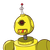# 9. The dimensions of a cuboidal box are in the ratio 3:2:1 and the total surface area is2,816 sq cm. Find its weight if dens

9. The dimensions of a cuboidal box are in the ratio 3:2:1 and the total surface area is
2,816 sq cm. Find its weight if density of the cuboidal box is 1 g/cm​

### 1 thought on “9. The dimensions of a cuboidal box are in the ratio 3:2:1 and the total surface area is<br />2,816 sq cm. Find its weight if dens”

1.Step-by-step explanation:

I think the weight should be 1g/cm³

Here, let

l= 3x

b= 2x and h= x

TSA= 2(lb+lh+bh)

2816cm²= 2(6x²+3x²+2x²)

2816cm²=2×11x²

256cm²=2x²

x= √256/2= 16/√2 = (8√2)cm

Vol³= l×b×h

= 3(8√2)cm ×2(8√2)cm×(8√2)cm

= 6cm³×512√8

=3072cm3×2√2= (6144√2)cm3= 8663.04cm3

therefore its weight= 8663.04cm³×1g/cm³= 8863.04g

or 8.86304 kg